# Texas Go Math Grade 7 Lesson 1.5 Answer Key Multiplying Rational Numbers

Refer to our Texas Go Math Grade 7 Answer Key Pdf to score good marks in the exams. Test yourself by practicing the problems from Texas Go Math Grade 7 Lesson 1.5 Answer Key Multiplying Rational Numbers.

## Texas Go Math Grade 7 Lesson 1.5 Answer Key Multiplying Rational Numbers

Question 1.
Use a number line to find 2(-35). __________Start at 0 Move 3.5 units to the left 2 times.
The result is -7.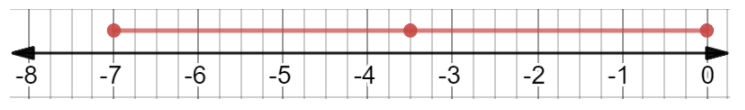Find -3(-1.25). __________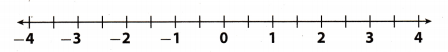First, find the product 3(-1.25).
Start at 0. Move 1.25 units to the left two times.
The result is -3.75.
This shows that 3 groups of -1.25 equals -3.75.
So, -3 groups of -1.25 must equal the opposite of -3.75.
-3(-1.25) = 3.75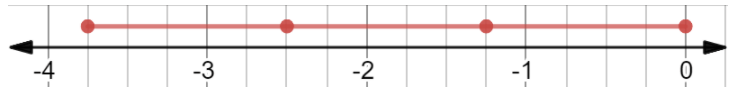Reflect

Question 3.
Look for a Pattern You know that the product of two negative numbers is positive, and the product of three negative numbers is negative. Write a rule for finding the sign of the product of n negative numbers.
The product of n negative numbers will be positive if n is even, or negative if n is odd.

Find each product.

Question 4.
(-$$\frac{3}{4}$$) (-$$\frac{4}{7}$$) (-$$\frac{2}{3}$$)
First, find the product of the first two factors. Both factors are negative, so their product will be positive.
(-$$\frac{3}{4}$$) (-$$\frac{4}{7}$$) = +($$\frac{3}{4}$$ . $$\frac{4}{7}$$)
= $$\frac{3}{7}$$
Now, multiply the result, which is positive, by the third factor, which is negative. The product will be negative
$$\frac{3}{7}$$ (-$$\frac{2}{3}$$) = $$\frac{3}{7}$$ (-$$\frac{2}{3}$$)
= –$$\frac{2}{7}$$

Question 5.
(-$$\frac{2}{3}$$) (-$$\frac{3}{4}$$) ($$\frac{4}{5}$$)
First, find the product of the first two factors Both factors are negative, so their product will be positive.
(-$$\frac{2}{3}$$) (-$$\frac{3}{4}$$) = + ($$\frac{2}{3}$$ . $$\frac{3}{4}$$)
= $$\frac{2}{4}$$
Now, multiply the result, which is positive, by the third factor, which is positive. The product will be positive.
$$\frac{2}{4}$$ ($$\frac{4}{5}$$) = $$\frac{2}{4}$$ ($$\frac{4}{5}$$) = $$\frac{2}{5}$$

Question 6.
($$\frac{2}{3}$$) (-$$\frac{9}{10}$$) ($$\frac{5}{6}$$)
First, find the product of the first two factors. The first factor is positive, second is negative, so their product will be negative.
($$\frac{2}{3}$$) (-$$\frac{9}{10}$$) = – ($$\frac{2}{3}$$ . $$\frac{9}{10}$$)
= –$$\frac{1}{2}$$
Now, multiply the result, which is negative, by the third factor, which is positive. The product will be negative.
–$$\frac{2}{5}$$ ($$\frac{5}{6}$$) = –$$\frac{2}{5}$$ ($$\frac{5}{6}$$) = –$$\frac{1}{3}$$

Use a number line to find each product. (Example 1 and Example 2)

Question 1.
5(-$$\frac{2}{3}$$) = _____________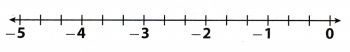Start at 0. Move $$\frac{2}{3}$$ to the left 5 times.
The result is –$$\frac{10}{3}$$.Question 2.
3(-$$\frac{1}{4}$$) = ____________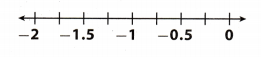Start at 0. Move $$\frac{1}{4}$$ to the left 5 times.
The result is –$$\frac{3}{4}$$.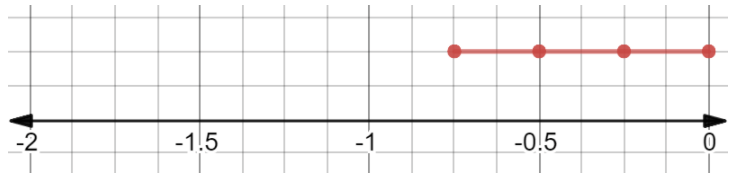-3(-$$\frac{4}{7}$$) = ____________First find the product 3(-4$$\frac{4}{7}$$).
Start at 0. Move $$\frac{4}{7}$$ units to the left 3 times.
The result is –$$\frac{12}{7}$$.
This shows that 3 groups of –$$\frac{4}{7}$$ equals –$$\frac{12}{7}$$.
So, -3 groups of –$$\frac{4}{7}$$ must equal to the opposite of –$$\frac{12}{7}$$.
-3(-$$\frac{4}{7}$$) = $$\frac{12}{7}$$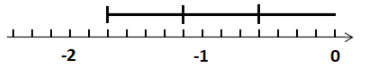Question 4.
–$$\frac{3}{4}$$ (-4) = ______________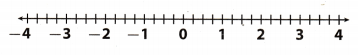Use the commutative property to swap multiplier and multiplicand.
-4(-$$\frac{3}{4}$$)
First, find the product 4(-$$\frac{3}{4}$$).
Start at 0. Move $$\frac{3}{4}$$ units to the left 4 times.
The result is -3.
This shows that 4 groups of –$$\frac{3}{4}$$ equals -3.
So, -4 groups of –$$\frac{3}{4}$$ must equal to the opposite of -3.
-4(-$$\frac{3}{4}$$) = 3Question 5.
4 (-3) = ___________
Start at 0. Move 3 to the left 4 times
The result is -12.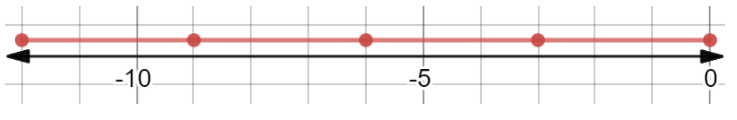Question 6.
-1.8 (5) = __________
Use commutative property to swap multiplier and multiplicand.
5(-1.8)
Start at 0. Move 1.8 to the left 5 times.
The result is -9.Go Math Grade 7 Lesson 1.5 Multiplying Rational Numbers Question 7.
-2 (-3.4) = ____________
First find the product 2(-3.4)
Start at 0. Move 3.4 units to the left 2 time&
The result is -6.8.
This shows that 2 groups of -3.4 equals -6.8.
So, -2 groups of -3.1 must equal to the opposite of -6.8.
-2(-3.4) = 6.8Question 8.
0.54 (8) = ___________
Given in problem : 0.54 (8) =
To find the product of 0.54 (8) on number line we will move 0.54 8 times on right of the number line because the product of 0.54 × 8 is positive.
The vaLue of: 0.54 × 8 = 4.32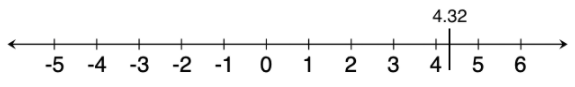Question 9.
-5 (- 1.2) = __________
First, find the product 5(-1.2).
Start at 0. Move 1.2 units to the left 5 times.
The result is -6.
This shows that 5 groups of -1.2 equals -6.
So, -5 groups of -1.2 must equal to the opposite of -6.
-5(-1.2) = 6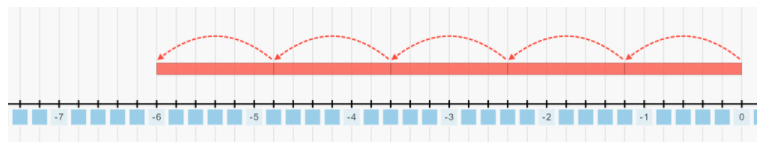Question 10.
-2.4 (3) = ____________
Use commutative property to swap multiplier and multiplicand.
3(-2.4)
Start at 0. Move 2.4 to the left 3 times.
The result is -7.2.Multiply. (Example 3)

Question 11.
$$\frac{1}{2}$$ × $$\frac{2}{3}$$ × $$\frac{3}{4}$$ = _________ × $$\frac{3}{4}$$ = __________
First, find the product of the first two factors. Both factors are positive, so their product will be positive.
$$\frac{1}{2}$$ × $$\frac{2}{3}$$ = $$\frac{1}{3}$$
Now, muLtiply the result, which is positive, by the third factor, which is positive. The product will be positive.
$$\frac{1}{3}$$ × $$\frac{3}{4}$$ = $$\frac{1}{4}$$

Question 12.
–$$\frac{4}{7}$$ (-$$\frac{3}{5}$$) (-$$\frac{7}{3}$$) = (__________) × (-$$\frac{7}{3}$$) = __________
First, find the product of the first two factors. Both factors are negative, so their product wiLl be positive
-($$\frac{4}{7}$$) (-$$\frac{3}{5}$$) = $$\frac{12}{35}$$
Now, muLtiply the resuLt, which is positive, by the third factor, which is negative. The product wilL be negative.
($$\frac{12}{35}$$) (-$$\frac{7}{3}$$) = ($$\frac{12}{35}$$) × (-$$\frac{7}{3}$$) = –$$\frac{4}{5}$$

Question 13.
–$$\frac{1}{8}$$ × 5 × $$\frac{2}{3}$$ = __________
First find the product of the first two factor & First factor is negative, second is positive, so their product will be negative.
–$$\frac{1}{8}$$ × 5 × $$\frac{2}{3}$$ = –$$\frac{5}{8}$$
Now, multiply the result, which is negative, by the third factor, which is positive. The product will be negative.
– $$\frac{5}{8}$$ × $$\frac{2}{3}$$ = –$$\frac{5}{12}$$

Go Math Grade 7 Lesson 1.5 Answer Key Rational Numbers Question 14.
–$$\frac{2}{3}$$ ($$\frac{1}{2}$$) (-$$\frac{6}{7}$$) = ___________
First, find the product of the first two factors. The first factor is negative, second is positive, so their product will be negative.
(-$$\frac{2}{3}$$) ($$\frac{1}{2}$$) = –$$\frac{1}{3}$$
Now, multiply the result, which is negative, by the third factor, which is negative. The product will be positive.
(-$$\frac{1}{3}$$) (-$$\frac{6}{7}$$) = $$\frac{2}{7}$$

Question 15.
The price of one share of Acme Company declined $3.50 per day for 4 days in a row. What is the overall change in the price of one share? (Example 1) Answer: Use negative number to represent the drop in price. Find 4(-3.50). Start at 0. Move 3.50 units to the left 4 times The result is -14. The overall change in the price of one share is -$14.Question 16.
In one day, 18 people each withdrew $100 from an ATM machine. What is the overall change in the amount of money in the ATM machine? (Example 1) Answer: Use a negative number to represent money withdrawn from an ATM machine. Find 18(-100). Start at 0. Move 100 units to the left 18 times The result is -1800 The overall change in the amount of money in the ATM machine is -$1800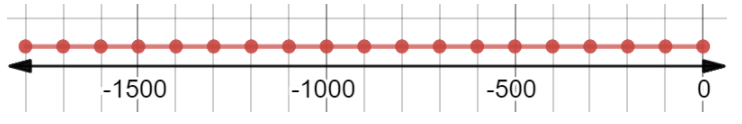Essential Question Check-In

Explain how you can find the sign of the product of two or more rational numbers.
If we have an even number of factors with a negative sign, the product will be positive.
If we have an odd number of factors with a negative sign, the product will be negative.

Question 18.
Financial Literacy Sandy has $200 in her bank account. a. If she writes 6 checks for exactly$19.98, what expression describes the change in her bank account?
Use a negative number to represent money lost with checks.
6(- 19.98)

b. What is her account balance after the checks are cashed?
We need to calculate the expression from a. and then subtract it from 200.
6(-19.98) = -119.88
200 – 119.88 = 80.12
Her account balance after the checks are cashed is \$80.12

Question 19.
Communicating Mathematical Ideas Explain, in words, how to find the product of -4(-1.5) using a number line. Where do you end up?
First, find the product 4(-1.5).
Start at 0. Move 1.5 units to the left 4 times.
The result is -6.
This shows that 4 groups of -1.5 equal -6.
So, -4 groups of -1.5 must equal to the opposite of -6.
-4(-1.5) = 6Question 20.
Greg sets his watch for the correct time on Wednesday. Exactly one week later, he finds that his watch has lost 3~ minutes. What is the overall change in time after 8 weeks?
Time Lost by Greg’s watch in 1 week = 3$$\frac{1}{4}$$ min
Time Lost by watch in 8 weeks = 8 × Time lest in 1 week
= 8 × 3$$\frac{1}{4}$$
= 8 × $$\frac{13}{4}$$
= 26 min
Hence, the time lost in 8 weeks will be 26 minutes.

Go Math 7th Grade Lesson 1.5 Multiplying Rational Numbers Answers Key Question 21.
A submarine dives below the surface, heading downward in three moves. If each move downward was 325 feet, where is the submarine after it is finished diving?
Use a negative number to represent the drop ¡n depth.
Find 3(-325).
Start at 0. Move 325 units to the left 3 times.
The result is -975.
The submarine after finishing diving is at -975 feet.Question 22.
Multistep For Home Economics class, Sandra has 5 cups of flour. She made 3 batches of cookies that each used 1.5 cups of flour. Write and solve an expression to find the amount of flour Sandra has left after making the 3 batches of cookies.
We need to calculate how many cups of flour she used on 3 batches of cookies, and then subtract it from how many cups of flour she has, which is 5.
5 – 3(1.5)
First find 3(1.5).
Start at 0. Move 1.5 units to the right 3 times.
The result is 4.5.
Sandra used 4.5 cups of flour for 3 batches of cookies.
5 – 3(1.5) = 5 – 4.5
= 0.5
Sandra has 0.5 batches of flour left.Question 23.
Critique Reasoning In class, Matthew stated, “I think that a negative is like an opposite. That is why multiplying a negative times a negative equals a positive. The opposite of negative is positive, so it is just like multiplying the opposite of a negative twice, which is two positives.” Do you agree or disagree with this statement? What would you say in response to him?
I agree with his statement
Let p. q be rational Opposite of p is q if p = -q. So negative q is the opposite of p

Question 24.
Kaitlin is on a long car trip. Every time she stops to buy gas, she loses 15 minutes of travel time. If she has to stop 5 times, how late will she be getting to her destination?
Use negative number to represent loss of minutes.
Find 5(-15).
Start at 0. Move 15 units to the left 5 times.
The result is -75.
Kaitlin lost 75 minutes, that means she will be 75 minutes late.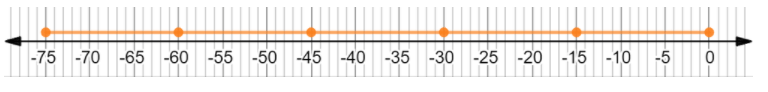Question 25.
The table shows the scoring system for quarterbacks in Jeremy’s fantasy football league. In one game, Jeremy’s quarterback had 2 touchdown passes, 16 complete passes, 7 incomplete passes, and 2 interceptions. How many total points did Jeremy’s quarterback score?Start by writing the expression of Jeremy’s quarterback using the table.
2(6) + 16(0.5) + 7(-0.5) + 2(-1.5) = 12 + 8 – 3.5 – 3
= 20 – 3.5 – 3
= 16.5 – 3
= 13.5
Jeremy’s quarterback scored 13.5 points.

H.O.T. Focus on Higher Order Thinking.

Question 26.
Represent Real-World Problems The ground temperature at Brigham Airport is 12 °C. The temperature decreases by 6.8 °C for every increase of 1 kilometer above the ground. What is the temperature outside a plane flying at an altitude of 5 kilometers?
Ground temperature of Brigham Airport = 12°
Height of the plane flying above ground = 5 Km
It is given in the problem that for every increase in 1 km above the ground the temperature decreases by 68°
So, the decrease in temperature will be 5 × 6.8 = 340
Temperature outside the plane = 12 – 34 = 220
Hence, the temperature outside the plane which is flying at an altitude of 5 kilometers will be -22°.

Multiplication Patterns Lesson 1.5 Go Math Answer Key Grade 7 Question 27.
Identify Patterns The product of four numbers, a, b, c, and d, is a negative number. The table shows one combination of positive and negative signs of the four numbers that could produce a negative product. Complete the table to show the seven other possible combinations.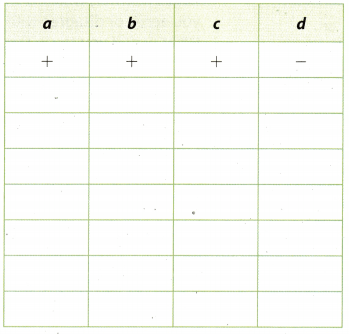We need to have an odd number of negative signs in a row.
Put +/— accordingly.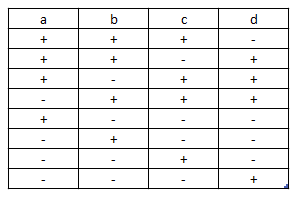Question 28.
Reason Abstractly Find two integers whose sum is -7 and whose product is 12. Explain how you found the numbers.